A simple Arduino/ESP32 crawler with PS4 BT remote control, but with 2x MCU and an advanced CAN-based communication ("drive-by-wire").

IntermediateWork in progress3,323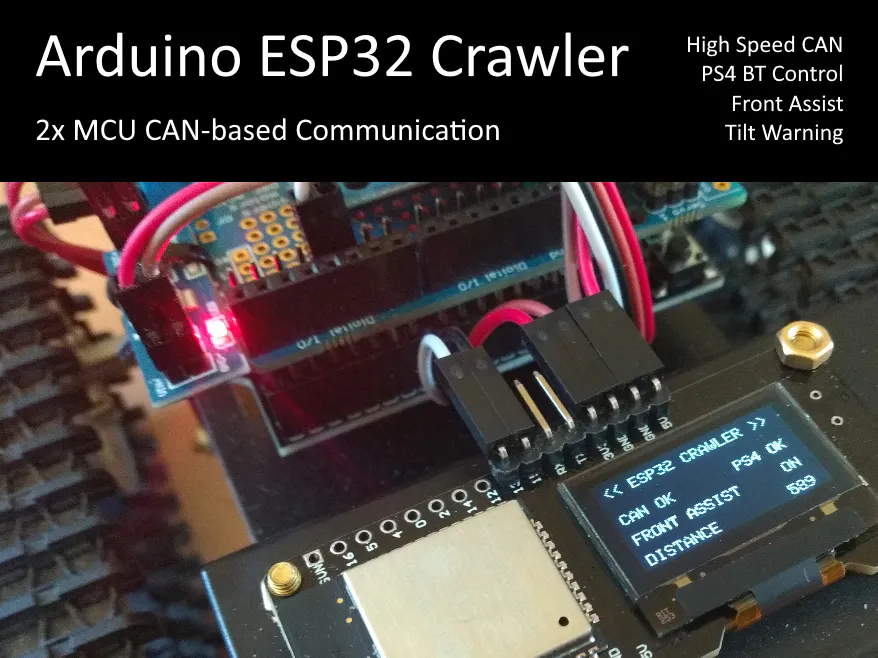## Things used in this project

### Hardware components

 DOIT T101 Mini
×1
 Wemos D1 R32 TT-GO variant uses Micro-USB instead of type A on Wemos variant.
×1
×1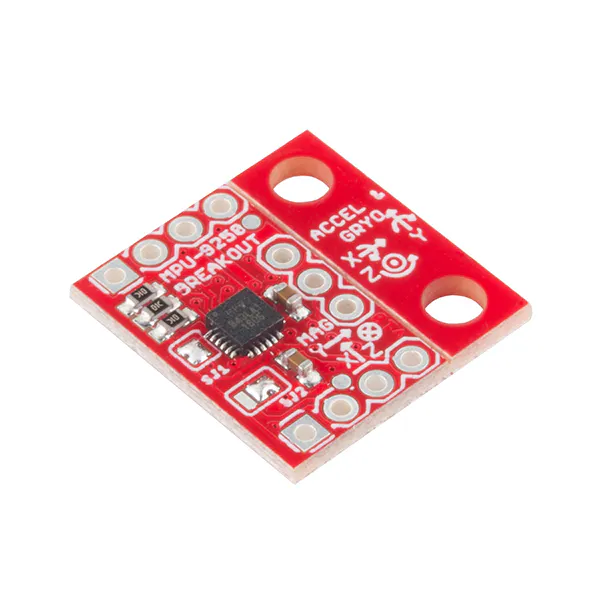SparkFun IMU Breakout - MPU-9250 9-DOF IMU
×1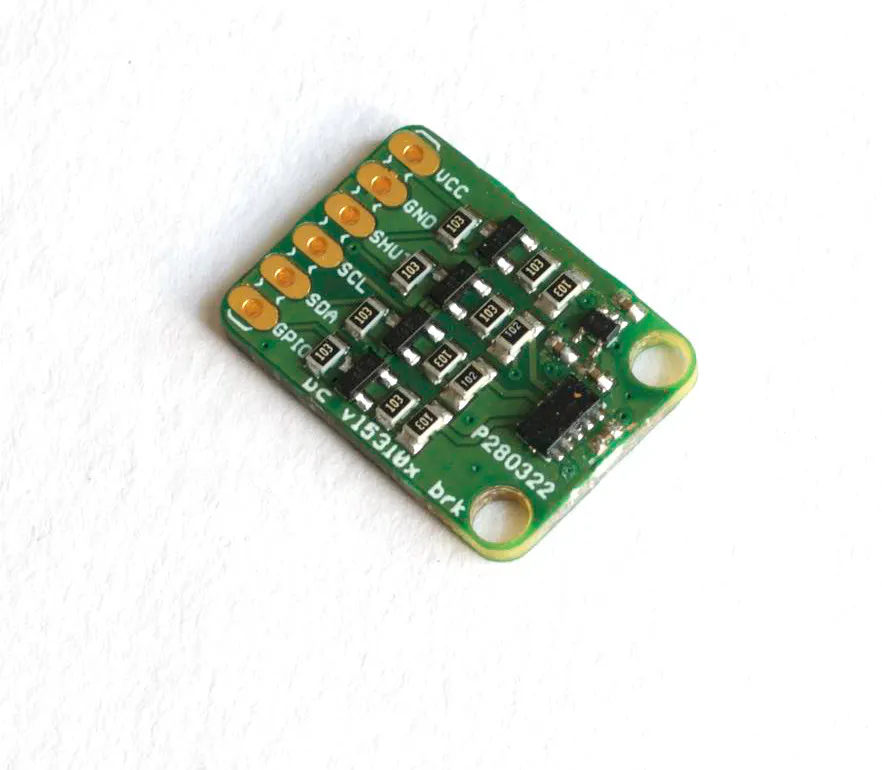ProtoCentral VL53L0X Laser ToF Sensor breakout board
×1
 D-duino32
×1
 AMS1117 5.0V
×1
 SN65HVD230 HS CAN Transceiver
×2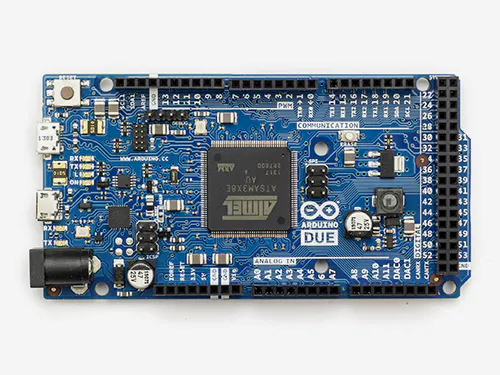Arduino Due Used as CAN monitor
×1
 SN65HVD230 Connected to Arduino Due
×1Jumper wires (generic)
×1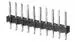Male Header 40 Position 1 Row (0.1")
×1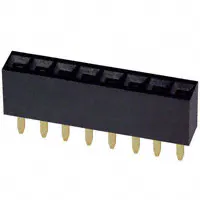Female Header 8 Position 1 Row (0.1")
×5

### Software apps and online servicesArduino IDE
 PuTTY

### Hand tools and fabrication machinesSoldering iron (generic)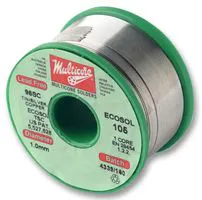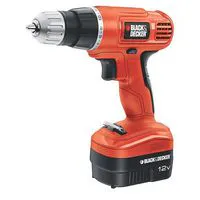Drill / Driver, Cordless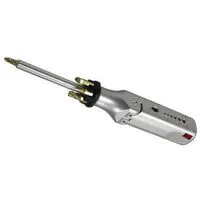Multitool, Screwdriver

## Code

### Wemos-D1-R32_Tank_T101_CAN_VL53L0X_MPU9250.ino

Arduino
```// << ESP32 Crawler >>
// board is "ESP32 Dev Module"
// CAN Driver https://github.com/nhatuan84/esp32-can-protocol-demo
// VL53L0X Driver from Pololu via Library Manager
// MPU9250 Driver from Bolder Flight System via Library Manager
// Adafruit Motor Shield V2 via Library Manager

#define LED_PIN 2

#include <Wire.h>

#define SPEED_MIN 70 // motor min
#define SPEED_MAX 255 // max speed
#define SPEED_NONE 0
int constSpeeds[] = {100, 150, 200, 250};
int speedIndex = 1;
bool incState = false;
bool incLast = false;
bool decState = false;
bool decLast = false;

#include <VL53L0X.h>
VL53L0X TOF;
#define TOF_INTERVALL 50 // ms
unsigned long lastTOFmeasurement = 0;

#define DISTANCE_MIN 170
int distance = 0;
bool auto_break  = true;
bool break_active = false;

#include "MPU9250.h"
MPU9250 IMU(Wire, 0x68);
#define TILT_MAX 45
bool tilt_warn = false;

#include <ESP32CAN.h>
#define CAN_SPEED CAN_SPEED_250KBPS
#define CAN_TX GPIO_NUM_16
#define CAN_RX GPIO_NUM_17
#include <CAN_config.h>

CAN_device_t CAN_cfg;
const int rx_queue_size = 10;

#define STICK_CAN_TIMEOUT 250
unsigned long lastStickReveiced;

int stick_left_x = 0;
int stick_left_y = 0;
int stick_right_x = 0;
int stick_right_y = 0;

bool button_left = false;
bool button_right = false;
bool button_up = false;
bool button_down = false;

bool button_square = false;
bool button_circle = false;
bool button_triangle = false;
bool button_cross = false;

bool button_l1 = false;
bool button_r1 = false;

for(;;) {
CAN_frame_t rx_frame;

if(rx_frame.FIR.B.FF==CAN_frame_std) { // standard frame format
digitalWrite(LED_PIN, HIGH);
// start of message evaluation
if (rx_frame.MsgID == 0x10 && rx_frame.FIR.B.DLC == 8) {
if (rx_frame.data.u8) {
stick_right_x = int(rx_frame.data.u8);
}
else {
stick_right_x = int(-(rx_frame.data.u8 + 1));
}
if (rx_frame.data.u8) {
stick_right_y = int(rx_frame.data.u8);
}
else {
stick_right_y = int(-(rx_frame.data.u8 + 1));
}
if (rx_frame.data.u8) {
stick_left_x = int(rx_frame.data.u8);
}
else {
stick_left_x = int(-(rx_frame.data.u8 + 1));
}
if (rx_frame.data.u8) {
stick_left_y = int(rx_frame.data.u8);
}
else {
stick_left_y = int(-(rx_frame.data.u8 + 1));
}
lastStickReveiced = millis();
}
else if (rx_frame.MsgID == 0x11 && rx_frame.FIR.B.DLC == 8) {
button_right = (bool)rx_frame.data.u8;
button_left = (bool)rx_frame.data.u8;
button_up = (bool)rx_frame.data.u8;
button_down = (bool)rx_frame.data.u8;
button_square = (bool)rx_frame.data.u8;
button_circle = (bool)rx_frame.data.u8;
button_triangle = (bool)rx_frame.data.u8;
button_cross = (bool)rx_frame.data.u8;
}
else if (rx_frame.MsgID == 0x12 && rx_frame.FIR.B.DLC == 8) {
button_r1 = (bool)rx_frame.data.u8;
button_l1 = (bool)rx_frame.data.u8;
}
// end of message evaluation
}
}
else {
digitalWrite(LED_PIN, LOW);
}
}
}

for(;;) {
CAN_frame_t tx_frame;
int d = distance;

tx_frame.FIR.B.FF==CAN_frame_std;
tx_frame.MsgID = 0x20;
tx_frame.FIR.B.DLC = 8;
tx_frame.data.u8 = byte(auto_break);
tx_frame.data.u8 = byte(break_active);
tx_frame.data.u8 = byte(tilt_warn);
tx_frame.data.u8 = 0;
tx_frame.data.u8 = byte(d>>(3*8));
tx_frame.data.u8 = byte(d<<(1*8)>>(3*8));
tx_frame.data.u8 = byte(d<<(2*8)>>(3*8));
tx_frame.data.u8 = byte(d<<(3*8)>>(3*8));

ESP32Can.CANWriteFrame(&tx_frame);

delay(50);
}
}

void setup()
{
//  Serial.begin(57600);

pinMode(LED_PIN, OUTPUT);
digitalWrite(LED_PIN, HIGH);

Wire.begin();

AFMS.begin();

right_motor->setSpeed(SPEED_NONE);
right_motor->run(RELEASE);

left_motor->setSpeed(SPEED_NONE);
left_motor->run(RELEASE);

TOF.setTimeout(500);
if (!TOF.init()) {
//    Serial.println("TOF init failed!");
while (1) {
digitalWrite(LED_PIN, LOW);
delay(500);
digitalWrite(LED_PIN, HIGH);
delay(500);
}
}
TOF.startContinuous();

if (IMU.begin() < 0) {
//    Serial.println("IMU init failed!");
while (1) {
digitalWrite(LED_PIN, LOW);
delay(1000);
digitalWrite(LED_PIN, HIGH);
delay(1000);
}
}

CAN_cfg.speed=CAN_SPEED;
CAN_cfg.tx_pin_id = CAN_TX;
CAN_cfg.rx_pin_id = CAN_RX;
CAN_cfg.rx_queue = xQueueCreate(rx_queue_size, sizeof(CAN_frame_t));

ESP32Can.CANInit();

digitalWrite(LED_PIN, LOW);

4096,      /* Stack size in words */
NULL,      /* Task input parameter */
1,         /* Priority of the task */
1);        /* Core where the task should run */

4096,      /* Stack size in words */
NULL,      /* Task input parameter */
1,         /* Priority of the task */
1);        /* Core where the task should run */

lastStickReveiced = millis();
}

void loop()
{
//  Serial.print("R_X: "); Serial.print(stick_right_x, DEC);
//  Serial.print(" R_Y: "); Serial.print(stick_right_y, DEC);
//  Serial.print(" L_X: "); Serial.print(stick_left_x, DEC);
//  Serial.print(" L_Y: "); Serial.print(stick_left_y, DEC);
//  Serial.println();

float acX, acY, acZ;
float p, r;
int pitch = 0, roll = 0;

acX = IMU.getAccelX_mss();
acY = IMU.getAccelY_mss();
acZ = IMU.getAccelZ_mss();
p = atan(acX / sqrt((acY * acY) + (acZ * acZ)));
r = atan(acY / sqrt((acX * acX) + (acZ * acZ)));
pitch = int(p * 57.2958); // 180.0 / pi
roll = int(r * 57.2958); // 180.0 / pi
tilt_warn = max(abs(pitch), abs(roll)) > TILT_MAX;
//  Serial.print("IMUP: "); Serial.print(pitch);
//  Serial.print(" IMUR: "); Serial.print(roll);
//  Serial.println();

delay(10);

// read TOF only once per intervall
if (millis() - lastTOFmeasurement >= TOF_INTERVALL) {
if (TOF.timeoutOccurred() || distance > 8191) distance = 8190;
lastTOFmeasurement = millis();
delay(10);
}
//  Serial.print("DIS: "); Serial.print(distance); Serial.print(" ");
//  Serial.println();

if ((millis() - lastStickReveiced) < STICK_CAN_TIMEOUT) {

int throttle = stick_left_y; // -128 0 127
int steering = stick_right_x; // -128 0 127
int left_speed = 0, right_speed = 0;

if (throttle > 0) throttle +=1; // max out to 128
if (steering > 0) steering +=1; // max out to 128

if (button_triangle) {
auto_break = true;
}
else if (button_cross) {
auto_break = false;
}

// mix throttle and steering inputs to obtain left & right motor speeds
left_speed = ((throttle * SPEED_MAX / 128) + (steering * SPEED_MAX / 128));
right_speed = ((throttle * SPEED_MAX / 128) - (steering * SPEED_MAX / 128));

// cap speeds to max
left_speed = min(max(left_speed, -SPEED_MAX), SPEED_MAX);
right_speed = min(max(right_speed, -SPEED_MAX), SPEED_MAX);

// decrease/increase fixed speed for digital pad control)
decState = button_l1;
if(decState && decState != decLast) {
if (speedIndex > 0) speedIndex--;
}
decLast = decState;
incState = button_r1;
if(incState && incState != incLast) {
if (speedIndex < 3) speedIndex++;
}
incLast = incState;

if (button_right){
left_speed = constSpeeds[speedIndex];
right_speed = -constSpeeds[speedIndex];
}
else if (button_left){
left_speed = -constSpeeds[speedIndex];
right_speed = constSpeeds[speedIndex];
}
else if (button_up) {
left_speed = constSpeeds[speedIndex];
right_speed = constSpeeds[speedIndex];
}
else if (button_down){
left_speed = -constSpeeds[speedIndex];
right_speed = -constSpeeds[speedIndex];
}

if (abs(left_speed) < SPEED_MIN) left_speed = 0;
if (abs(right_speed) < SPEED_MIN) right_speed = 0;

if (auto_break) {
break_active = false;
if (distance < (DISTANCE_MIN * 1.5) && left_speed > (SPEED_MAX / 2) && right_speed > (SPEED_MAX / 2)) {
left_speed = left_speed / 2;
right_speed = right_speed / 2;
}
if (distance < DISTANCE_MIN && left_speed > 0 && right_speed > 0) {
left_speed = SPEED_NONE;
right_speed = SPEED_NONE;
break_active = true;
}
}

//    Serial.print("RSDP: "); Serial.print(right_speed);
//    Serial.print(" LSPD: "); Serial.print(left_speed);
//    Serial.println();

right_motor->setSpeed(abs(right_speed));
if (right_speed >= 0) right_motor->run(FORWARD);
else right_motor->run(BACKWARD);

left_motor->setSpeed(abs(left_speed));
if (left_speed >= 0) left_motor->run(FORWARD);
else left_motor->run(BACKWARD);

delay(10);
}
else {
//    Serial.println("TIMEOUT: Stick Frame not received");

stick_left_x = 0;
stick_left_y = 0;
stick_right_x = 0;
stick_right_y = 0;

button_left = false;
button_right = false;
button_up = false;
button_down = false;

button_square = false;
button_circle = false;
button_triangle = false;
button_cross = false;

button_l1 = false;
button_r1 = false;

right_motor->setSpeed(SPEED_NONE);
right_motor->run(RELEASE);

left_motor->setSpeed(SPEED_NONE);
left_motor->run(RELEASE);

delay(STICK_CAN_TIMEOUT/2);
}
}
```

### D-duino-32_Tank_T101_CAN_PS4_OLED.ino

Arduino
```// << ESP32 Crawler >>
// board is "D-duino-32"
// CAN Driver https://github.com/nhatuan84/esp32-can-protocol-demo
// PS4 Driver https://github.com/NURobotics/PS4-esp32
// PS4 MAC https://dancingpixelstudios.com/sixaxis-controller/sixaxispairtool/
// in case the controller is no longer pairing but was working before,
// run "esptool --chip esp32 erase_flash" in C:\Users\<user>\AppData\Local\Arduino15\packages\esp32\tools\esptool_py\2.6.1
// see https://github.com/NURobotics/PS4-esp32/issues/2#issuecomment-602852034

#include <Wire.h>
#include <Adafruit_SSD1306.h> // works only if board and variant config for D-duino-32 is available

#define SCREEN_WIDTH 128 // OLED display width, in pixels
#define SCREEN_HEIGHT 64 // OLED display height, in pixels

#define OLED_RESET 3
#define OLED_ROTATION 0

#if (SSD1306_LCDHEIGHT != 64)
#endif

#include <PS4Controller.h>
#define PS4_HOST_MAC "00:1e:ab:4c:4e:c8"
#define TILT_RUMBLE 127

#include <ESP32CAN.h>
#define CAN_SPEED CAN_SPEED_250KBPS
#define CAN_TX GPIO_NUM_15
#define CAN_RX GPIO_NUM_13
#include <CAN_config.h>

CAN_device_t CAN_cfg;
const int rx_queue_size = 10;

#define STATUS_CAN_TIMEOUT 250
unsigned long lastStatusReveiced;

bool auto_break = false;
bool break_active = false;
int distance = 0;
byte tilt_warn = false;

for(;;) {
CAN_frame_t rx_frame;

// start of message evaluation
if(rx_frame.FIR.B.FF==CAN_frame_std) { // standard frame format
if (rx_frame.MsgID == 0x20 && rx_frame.FIR.B.DLC == 8) {
auto_break =   bool(rx_frame.data.u8);
break_active = bool(rx_frame.data.u8);
tilt_warn =    bool(rx_frame.data.u8);
distance  =     int(rx_frame.data.u8)<<(3*8);
distance +=     int(rx_frame.data.u8)<<(2*8);
distance +=     int(rx_frame.data.u8)<<(1*8);
distance +=     int(rx_frame.data.u8);

lastStatusReveiced = millis();
}
}
// end of message evaluation
}
}
}

void setup()
{
setCpuFrequencyMhz(160); // reduce heat emission & power consumption (default would be 240Mhz)

//  Serial.begin(57600);

if(!display.begin(SSD1306_SWITCHCAPVCC, 0x3c)) { // 0x3c for on-board OLED of D-duino-32
//    Serial.println(F("SSD1306 allocation failed"));
while (1) {}
}
display.clearDisplay();
display.setTextSize(1);
display.setTextColor(WHITE, BLACK);
display.setCursor(5,5);
display.print("<< ESP32 CRAWLER >>");
display.display();

PS4.begin(PS4_HOST_MAC);

CAN_cfg.speed=CAN_SPEED;
CAN_cfg.tx_pin_id = CAN_TX;
CAN_cfg.rx_pin_id = CAN_RX;
CAN_cfg.rx_queue = xQueueCreate(rx_queue_size, sizeof(CAN_frame_t));

ESP32Can.CANInit();

4096,      /* Stack size in words */
NULL,      /* Task input parameter */
1,         /* Priority of the task */
1);        /* Core where the task should run */

lastStatusReveiced = 0;
}

void loop()
{
if(PS4.isConnected()) {

//    Serial.print("R_X: "); Serial.print(PS4.data.analog.stick.rx, DEC);
//    Serial.print(" R_Y: "); Serial.print(PS4.data.analog.stick.ry, DEC);
//    Serial.print(" L_X: "); Serial.print(PS4.data.analog.stick.lx, DEC);
//    Serial.print(" L_Y: "); Serial.print(PS4.data.analog.stick.ly, DEC);
//    Serial.println();

//    Serial.print("DIS: "); Serial.println(distance);

display.setCursor(83,25);
display.print("PS4 OK ");

display.setCursor(5, 40);
if (auto_break) {
if (break_active) {
display.print("FRONT ASSIST ACTIVE");
PS4.setLed(255, 0, 0);
}
else {
display.print("FRONT ASSIST     ON ");
PS4.setLed(0, 255, 0);
}
}
else {
display.print("FRONT ASSIST     OFF");
PS4.setLed(0, 0, 255);
}

#ifdef TILT_RUMBLE
if (tilt_warn) {
PS4.setRumble(0, TILT_RUMBLE);
}
else {
PS4.setRumble(0, 0);
}
#endif

// PS4.sendToController(); // moved after sending frames
// delay(15); // replaced by delay between frames

display.setCursor(5, 55);
display.print("DISTANCE");
char sf;
sprintf(sf, "%4d", distance);
display.setCursor(95, 55);
display.print(sf);

int rgt_stick_x = PS4.data.analog.stick.rx; // -128 0 127
int rgt_stick_y = PS4.data.analog.stick.ry; // -128 0 127
int lft_stick_x = PS4.data.analog.stick.lx; // -128 0 127
int lft_stick_y = PS4.data.analog.stick.ly; // -128 0 127

// prepare analog sticks (bytes)
byte b_rgt_stick_x_val;
byte b_rgt_stick_x_dir;
if (rgt_stick_x >= 0) {
b_rgt_stick_x_val = byte(rgt_stick_x);
b_rgt_stick_x_dir = 1;
}
else {
b_rgt_stick_x_val = byte(abs(rgt_stick_x)-1);
b_rgt_stick_x_dir = 0;
}

byte b_rgt_stick_y_val;
byte b_rgt_stick_y_dir;
if (rgt_stick_y >= 0) {
b_rgt_stick_y_val = byte(rgt_stick_y);
b_rgt_stick_y_dir = 1;
}
else {
b_rgt_stick_y_val = byte(abs(rgt_stick_y)-1);
b_rgt_stick_y_dir = 0;
}

byte b_lft_stick_x_val;
byte b_lft_stick_x_dir;
if (lft_stick_x >= 0) {
b_lft_stick_x_val = byte(lft_stick_x);
b_lft_stick_x_dir = 1;
}
else {
b_lft_stick_x_val = byte(abs(lft_stick_x)-1);
b_lft_stick_x_dir = 0;
}

byte b_lft_stick_y_val;
byte b_lft_stick_y_dir;
if (lft_stick_y >= 0) {
b_lft_stick_y_val = byte(lft_stick_y);
b_lft_stick_y_dir = 1;
}
else {
b_lft_stick_y_val = byte(abs(lft_stick_y)-1);
b_lft_stick_y_dir = 0;
}

CAN_frame_t tx_control;

// Send analog sticks bytes on CAN
tx_control.FIR.B.FF==CAN_frame_std;
tx_control.MsgID = 0x10;
tx_control.FIR.B.DLC = 8;
tx_control.data.u8 = b_rgt_stick_x_val;
tx_control.data.u8 = b_rgt_stick_x_dir;
tx_control.data.u8 = b_rgt_stick_y_val;
tx_control.data.u8 = b_rgt_stick_y_dir;
tx_control.data.u8 = b_lft_stick_x_val;
tx_control.data.u8 = b_lft_stick_x_dir;
tx_control.data.u8 = b_lft_stick_y_val;
tx_control.data.u8 = b_lft_stick_y_dir;

ESP32Can.CANWriteFrame(&tx_control);

delay(5);

// send button states as byte on CAN
tx_control.FIR.B.FF==CAN_frame_std;
tx_control.MsgID = 0x11;
tx_control.FIR.B.DLC = 8;
tx_control.data.u8 = byte(PS4.data.button.right);
tx_control.data.u8 = byte(PS4.data.button.left);
tx_control.data.u8 = byte(PS4.data.button.up);
tx_control.data.u8 = byte(PS4.data.button.down);
tx_control.data.u8 = byte(PS4.data.button.square);
tx_control.data.u8 = byte(PS4.data.button.circle);
tx_control.data.u8 = byte(PS4.data.button.triangle);
tx_control.data.u8 = byte(PS4.data.button.cross);
ESP32Can.CANWriteFrame(&tx_control);

delay(5);

// send button states as byte on CAN
tx_control.FIR.B.FF==CAN_frame_std;
tx_control.MsgID = 0x12;
tx_control.FIR.B.DLC = 8;
tx_control.data.u8 = byte(PS4.data.button.r1);
tx_control.data.u8 = byte(PS4.data.button.l1);
tx_control.data.u8 = byte(PS4.data.button.r2);
tx_control.data.u8 = byte(PS4.data.analog.button.r2);
tx_control.data.u8 = byte(PS4.data.button.l2);
tx_control.data.u8 = byte(PS4.data.analog.button.l2);
tx_control.data.u8 = byte(PS4.data.button.r3);
tx_control.data.u8 = byte(PS4.data.button.l3);
ESP32Can.CANWriteFrame(&tx_control);

delay(5);

PS4.sendToController(); // should not be done more than each 15ms, but with delay (5+5+5) from tx CAN should be ok
}
else {
//    Serial.println("No PS4 controller connected.");
display.setCursor(83,25);
display.print("PS4 NOK");
display.setCursor(5, 40);
display.print("                    ");
display.setCursor(5, 55);
display.print("                    ");
display.display();

// delay(5);

CAN_frame_t tx_control;

// Send analog sticks bytes on CAN
tx_control.FIR.B.FF==CAN_frame_std;
tx_control.MsgID = 0x10;
tx_control.FIR.B.DLC = 8;
tx_control.data.u8 = 0;
tx_control.data.u8 = 0;
tx_control.data.u8 = 0;
tx_control.data.u8 = 0;
tx_control.data.u8 = 0;
tx_control.data.u8 = 0;
tx_control.data.u8 = 0;
tx_control.data.u8 = 0;
ESP32Can.CANWriteFrame(&tx_control);

delay(5);

// send button states as byte on CAN
tx_control.FIR.B.FF==CAN_frame_std;
tx_control.MsgID = 0x11;
tx_control.FIR.B.DLC = 8;
tx_control.data.u8 = 0;
tx_control.data.u8 = 0;
tx_control.data.u8 = 0;
tx_control.data.u8 = 0;
tx_control.data.u8 = 0;
tx_control.data.u8 = 0;
tx_control.data.u8 = 0;
tx_control.data.u8 = 0;
ESP32Can.CANWriteFrame(&tx_control);

delay(5);

// send button states as byte on CAN
tx_control.FIR.B.FF==CAN_frame_std;
tx_control.MsgID = 0x12;
tx_control.FIR.B.DLC = 8;
tx_control.data.u8 = 0;
tx_control.data.u8 = 0;
tx_control.data.u8 = 0;
tx_control.data.u8 = 0;
tx_control.data.u8 = 0;
tx_control.data.u8 = 0;
tx_control.data.u8 = 0;
tx_control.data.u8 = 0;
ESP32Can.CANWriteFrame(&tx_control);

delay(5);
}
if ((millis() - lastStatusReveiced) < STATUS_CAN_TIMEOUT) {
display.setCursor(5,25);
display.print("CAN OK ");
}
else {
auto_break = false;
break_active = false;
distance = 0;
tilt_warn = false;
display.setCursor(5,25);
display.print("CAN NOK");
display.setCursor(5, 40);
display.print("                    ");
display.setCursor(5, 55);
display.print("                    ");
delay(15);
}
display.display();
}
```

## Credits

### srolf

0 projects • 0 followers